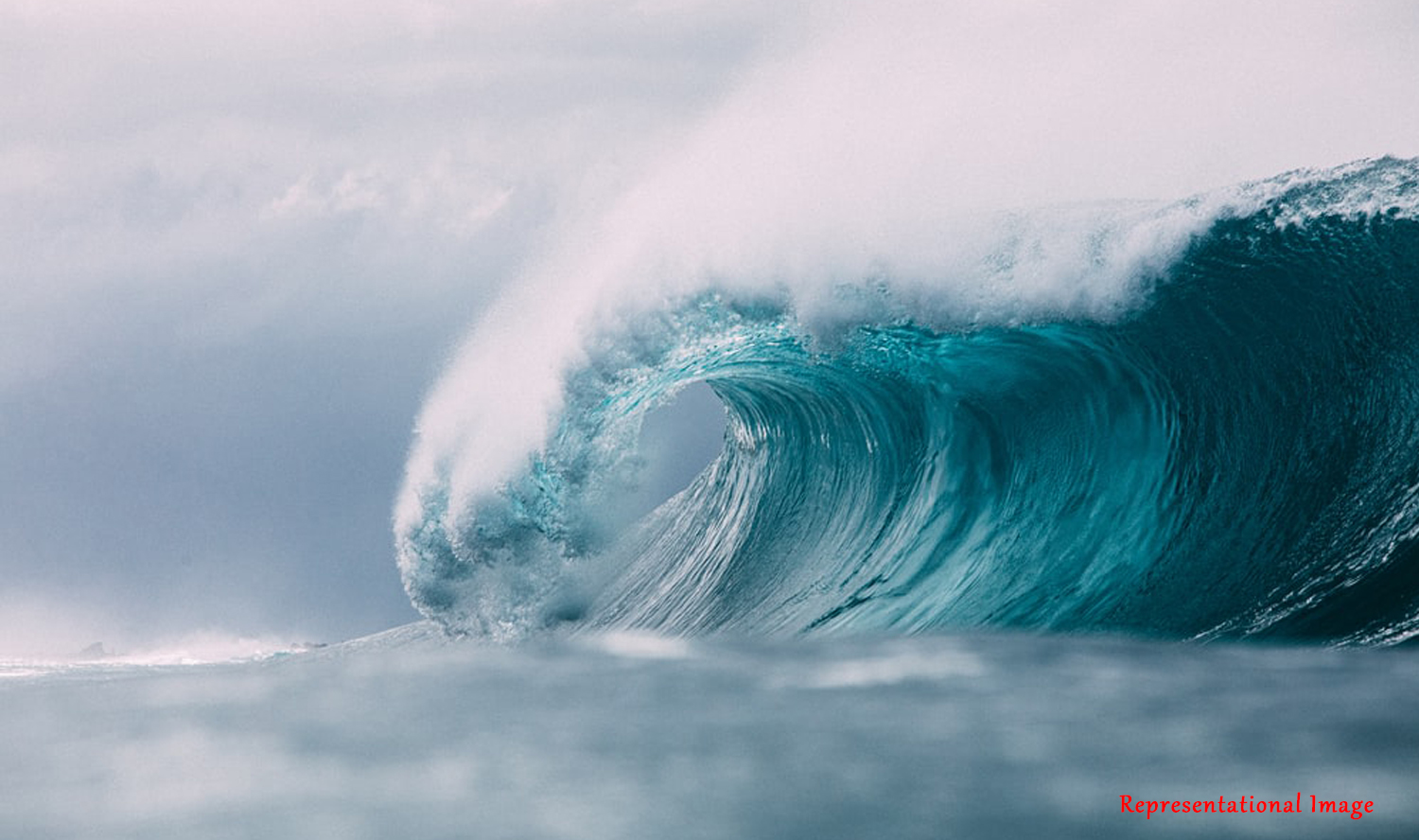Waves of Water and MathThe scattering of water waves at an angle on vertical porous barriers is of interest in the fields of coastal and ocean engineering. Interestingly, the solutions to the studies of scattering of water waves at an angle are solved by mathematics. The mathematics used in this case is the use of integral equations.

The mathematical method used to study the scattering problem is known as the Galerkin method.   In this study, Mr. R. Ashok, and Prof. Srinivasa Rao Manam, from the Department of Mathematics, IIT Madras, convert the original half-plane problems into a set of quarter-plane problems, by making a novel connection between involved potentials. The various barriers taken into consideration are as follows:

1. Surface piercing plate

2. Submerged barrier with infinite length

3. Complete barrier with a gap

4. Submerged barrier with finite length

Two solution potentials are utilized by allowing the waves from either side of the barrier. The original problem is decomposed into two resolvable boundary value problems in the quarter-plane. Single or two-term Galerkin approximation method is used to solve the decomposed problems and get closed bounds for the reflection and the transmission coefficients.

With the use of Galerkin approximation, it was found that enhanced reflection occurs at certain incident angles as well as at certain incident wave frequencies for all four types of vertical elastic porous barriers. It is noted that the results for inelastic solid or porous barriers match with the ones which are already available in the existing literature.

Prof. Birendra Nath Mandal, retired professor and NASI Senior Scientist Platinum Jubilee Fellow (2009-2014) of the Physics and Applied Mathematics Unit, Indian Statistical Institute, Kolkata, gave the following critical comments on the paper: “The study on water wave scattering by thin vertical barriers with various configurations is of interest in the field of coastal and ocean engineering. In the present paper, scattering of obliquely incident water waves by various vertical elastic porous barrier configurations is investigated. The original problem is decomposed into two boundary value problems which are solved by applying single or two-term Galerkin approximation method to get very accurate numerical estimates for the reflection and the transmission coefficients, which are depicted graphically in a number of figures.”

Article by Akshay Anantharaman
Here is the original link to the paper:
https://www.sciencedirect.com/science/article/pii/S0020722521001208?dgcid=coauthor

.